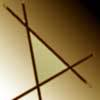Search by Topic

Resources tagged with Solving equations graphically similar to Which Is Bigger?:

Filter by: Content type:
Age range:
Challenge level:

There are 5 results

Broad Topics > Functions and Graphs > Solving equations graphicallyWhich Is Bigger?

Age 14 to 16 Challenge Level:

Which is bigger, n+10 or 2n+3? Can you find a good method of answering similar questions?Which Is Cheaper?

Age 14 to 16 Challenge Level:

When I park my car in Mathstown, there are two car parks to choose from. Can you help me to decide which one to use?Negatively Triangular

Age 14 to 16 Challenge Level:

How many intersections do you expect from four straight lines ? Which three lines enclose a triangle with negative co-ordinates for every point ?Intersections

Age 14 to 18 Challenge Level:

Change one equation in this pair of simultaneous equations very slightly and there is a big change in the solution. Why?Temperature

Age 11 to 14 Challenge Level:

Is there a temperature at which Celsius and Fahrenheit readings are the same?